# Tiling & packing problems

#### Example 1

Paint is sold in 4 litre tins. If I need 17 litres of paint, how many tins will I need to buy?

Solution

17 litres ÷ 4 litres = 4 remainder 1

So, I must buy 5 tins of paint to have at least 17 litres, even though most of the last tin of paint will be left over.

This is an example where I need to overestimate since I can only buy whole tins.

#### Example 2

I am considering tiling the floor in my kitchen which measures 8.9m by 12.4m. The tiles I have chosen measure 1m by 1m, and have a design on them so they can not be turned round. How many tiles will be required?

Solution

Along the width I will require 9 tiles

Along the length I will require 13 tiles
so, to cover the whole kitchen I will need 9 × 13 = 117 tiles

This is another example of when we need to overestimate. The actual area of the floor is less than 117 square metres. There will be parts of tiles left over which we will not use.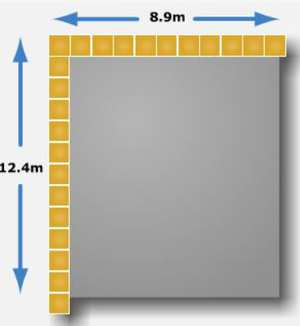#### Example 3

A factory makes tiles each measuring 8cm by 6cm. The boxes to transport these have a base which measures 34cm by 28cm. The tiles must be laid flat for transport. Which is the most efficient way to arrange the tiles in the box?

Solution

There are 2 possible ways of arranging the tiles:

Arrangement 1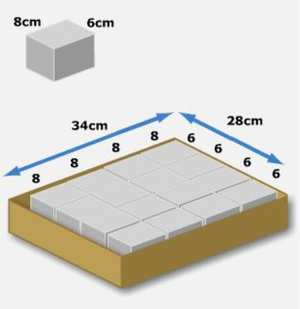Imagine we arrange the boxes with the 6cm edge along the width and the 8cm edge along the length.

How many boxes can we fit along the width?
We can fit 28 ÷ 6 = 4.67 i.e. 4 boxes.

How many boxes can we fit along the length?
We can fit 34 ÷ 8 = 4.25 i.e. 4 boxes.

This gives a total of 4×4 = 16 boxes.

Arrangement 2Now imagine we arrange the boxes with the 8cm edge along the width and the 6cm edge along the length.

How many boxes can we fit along the width?
We can fit 28 ÷ 8 = 3.5 i.e. 3 whole boxes

How many boxes can we fit along the length?
We can fit 34 ÷ 6 = 5.67 i.e. 5 whole boxes

This gives a total of 5×3 = 15 boxes.

So Arrangement 1 is the more efficient way to arrange the tiles. We can fit 16 tiles on the base this way as opposed to 15 tiles if the tiles are orientated the other way.

In this example, we had to underestimate since we can only sell whole tiles. There will be space left over in the box which we can not fill.

#### Example 4

Boxes of chocolate measure 7cm × 10cm × 4cm. These must be packaged into crates with a base measuring 75cm × 60cm and a height of 50cm. What is the most efficient way to package the chocolate boxes into crates?

Solution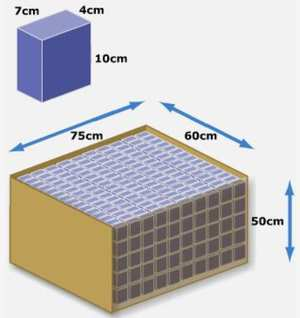Arrangement 1

If we arrange the boxes with the 10cm side upwards, this would mean we have
50 ÷ 10 = 5 layers

There would then be two ways we could arrange the boxes on each layer:

Either

The 4 cm edge along the width so
60 ÷ 4 = 15 boxes

and

the 7cm edge along the length so

75 ÷ 7 = 10.71 i.e. 10 boxes

This gives a total of 5×15 × 10 = 750 boxes.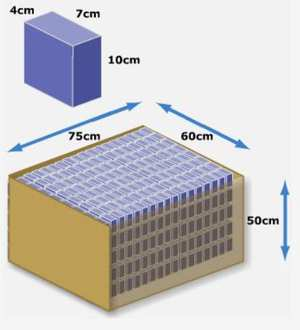or

The 7 cm edge along the width so
60 ÷ 7 = 8.6 i.e 8 boxes.

and

the 4cm edge along the length so
75 ÷ 4 = 18.75 i.e. 18 boxes.

giving a total of 5×8 × 18 =720 boxes.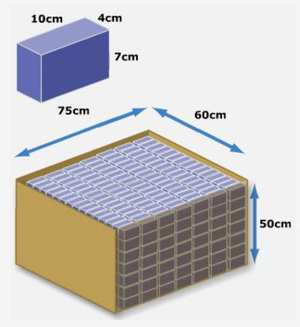Arrangement 2

If we arrange the boxes with the 7cm side upwards, this would mean we have
50 ÷ 7 = 7.1 i.e. 7 layers

There would then be two ways we could arrange the boxes on each layer:

Either

The 4 cm edge along the width so
60 ÷ 4 = 15 boxes.

and

the 10cm edge along the length so
75 ÷ 10 = 7.5 i.e 7 boxes.

This gives a total of 7×15 × 7 =735 boxes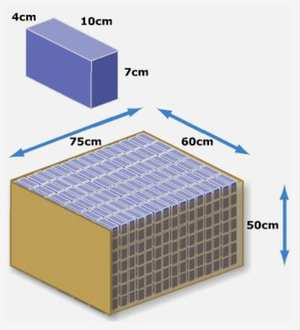or

The 10 cm edge along the width so
60 ÷ 10 = 6 boxes.

and

the 4cm edge along the length so

75 ÷ 4 = 18.75 i.e 18 boxes.

This gives a total of 7×6 × 18 =756 boxes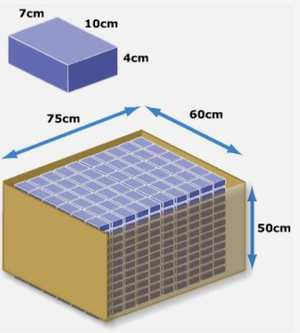Arrangement 3

If we arrange the boxes with the 4cm side upwards, this would mean we have
50 ÷ 4 = 12.5 i.e. 12 layers

There would then be two ways we could arrange the boxes on each layer:

Either

The 10 cm edge along the width so
60 ÷ 10 = 6 boxes exactly.

and

7cm edge along the length so
75 ÷ 7 = 10.7 i.e. 10 boxes.

This gives a total of 12×6 × 10 =720 boxes.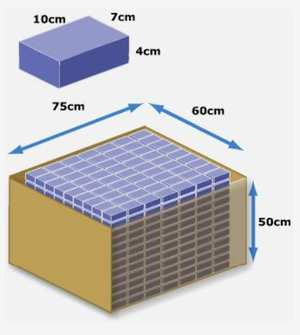or

7 cm edge along the width so
60 ÷ 7 = 8.75 i.e. 8 boxes.

and 10cm along the length so
75 ÷ 10 = 7.5 i.e. 7 boxes.

This gives a total of 12 × 8 × 7 = 672 boxes.

So the solution which allows the most boxes to be packed into a crate is Arrangement 2 with 7cm upwards, 4cm along the length of the base, and 10cm along the width. This gives a total of 756 boxes in the crate.

Again we must underestimate for this problem. There will be some space left over in the crate which we cannot fill with chocolate boxes. The best solution is the one with the least space left empty!